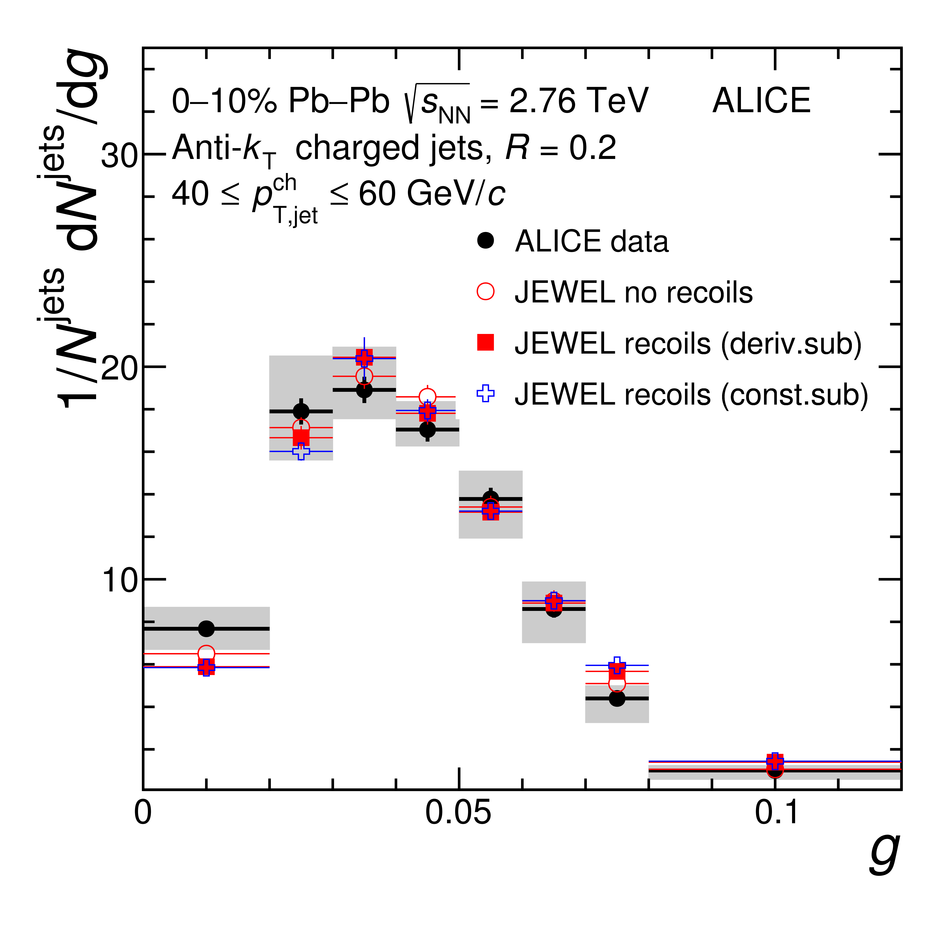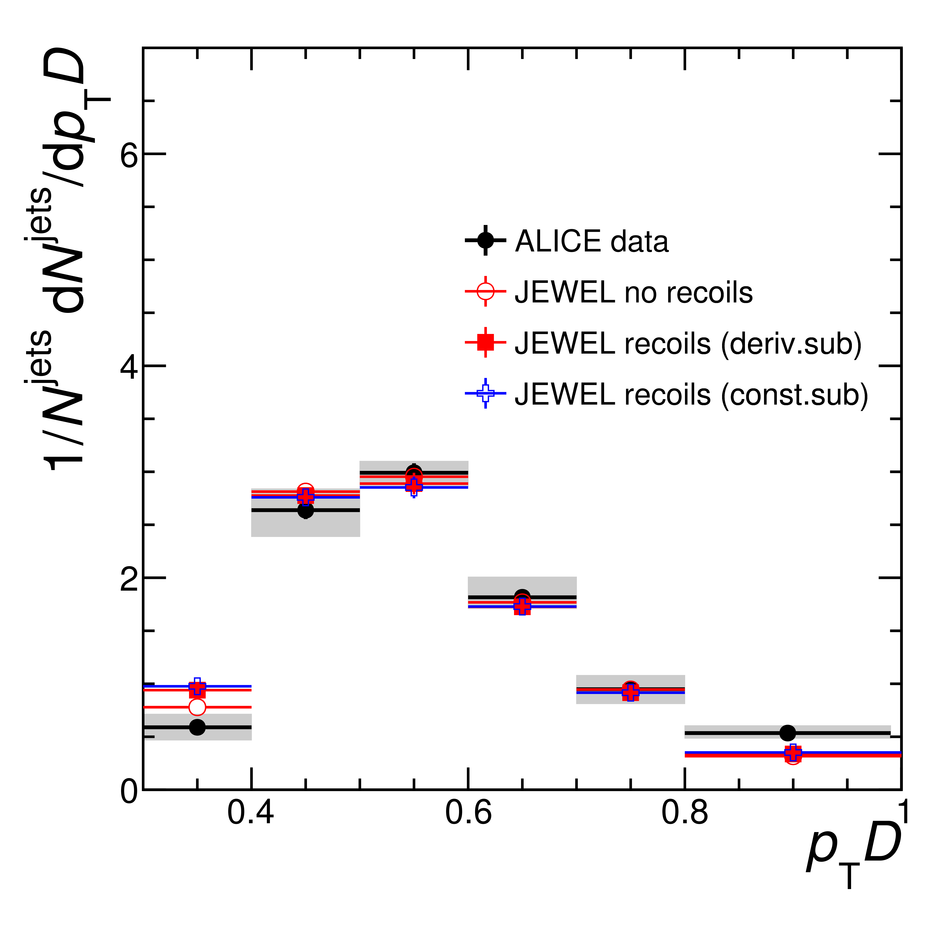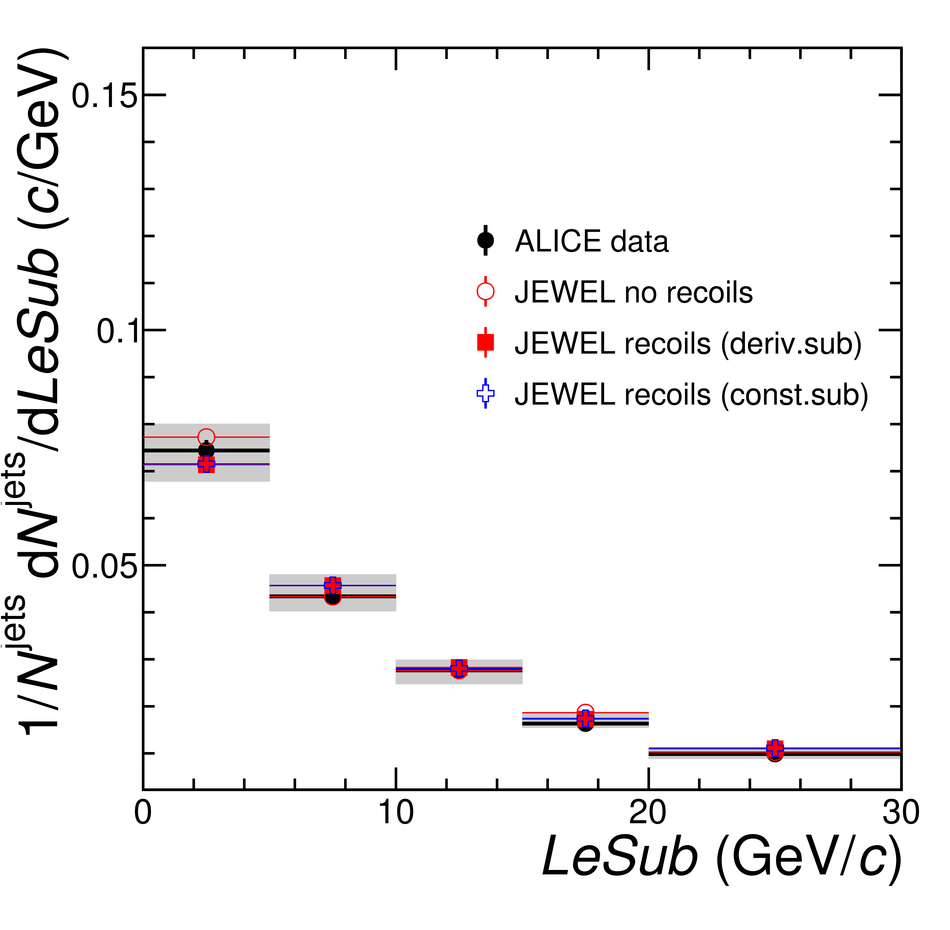# Medium modification of the shape of small-radius jets in central Pb-Pb collisions at $\sqrt{s_{\mathrm {NN}}} = 2.76\,\rm{TeV}$

We present the measurement of a new set of jet shape observables for track-based jets in central Pb-Pb collisions at $\sqrt{s_{\mathrm{NN}}} = 2.76$ TeV. The set of jet shapes includes the first radial moment or angularity, $g$; the momentum dispersion, $p_{\rm T}D$; and the difference between the leading and sub-leading constituent track transverse momentum, $LeSub$. These observables provide complementary information on the jet fragmentation and can constrain different aspects of the theoretical description of jet-medium interactions. The jet shapes were measured for a small resolution parameter $R = 0.2$ and were fully corrected to particle level. The observed jet shape modifications indicate that in-medium fragmentation is harder and more collimated than vacuum fragmentation as obtained by PYTHIA calculations, which were validated with the measurements of the jet shapes in proton-proton collisions at $\sqrt{s} = 7$ TeV. The comparison of the measured distributions to templates for quark and gluon-initiated jets indicates that in-medium fragmentation resembles that of quark jets in vacuum. We further argue that the observed modifications are not consistent with a totally coherent energy loss picture where the jet loses energy as a single colour charge, suggesting that the medium resolves the jet structure at the angular scales probed by our measurements ($R=0.2$). Furthermore, we observe that small-$R$ jets can help to isolate purely energy loss effects from other effects that contribute to the modifications of the jet shower in medium such as the correlated background or medium response.

Figures

## Figure 1

 $g$, $p_{\rm T}D$, and $LeSub$ for quark and gluon jets as obtained from PYTHIA Perugia 2011 simulations of pp collisions at $\sqrt{s}=2.76$\,TeV in the transverse momentum interval $40 \leq p_{\mathrm{T,jet}}^{\rm{part,ch}} \leq 60$\,GeV/$c$.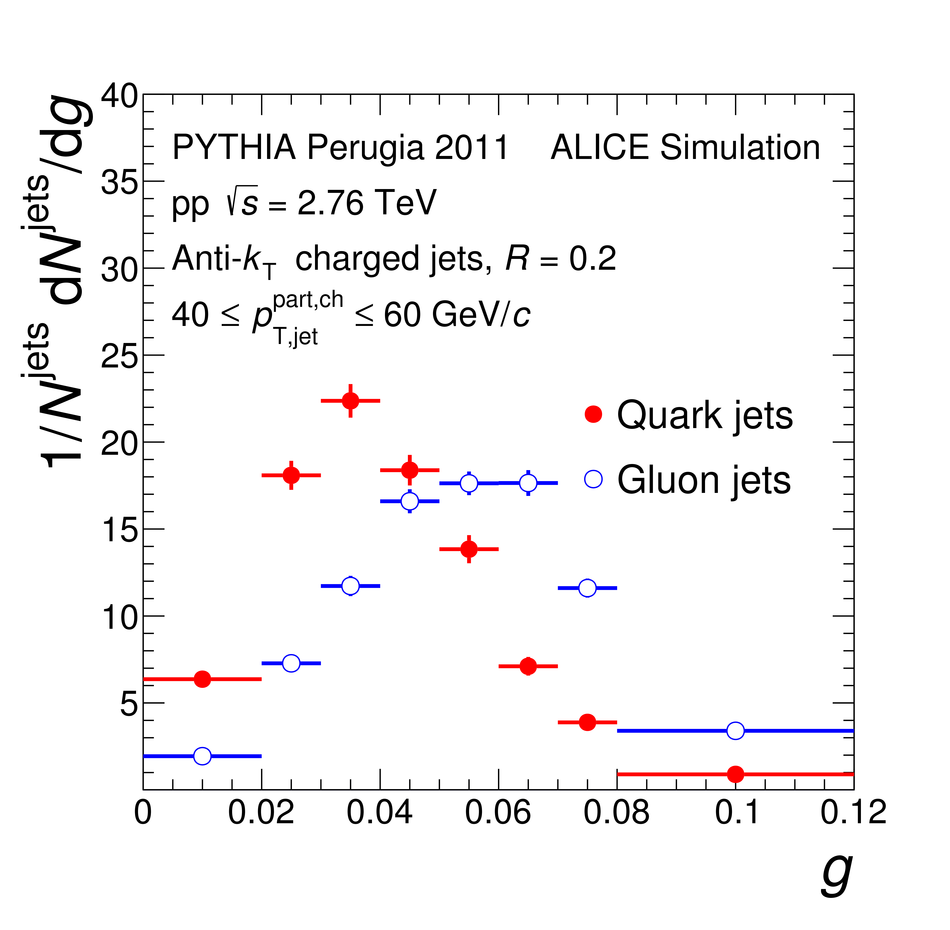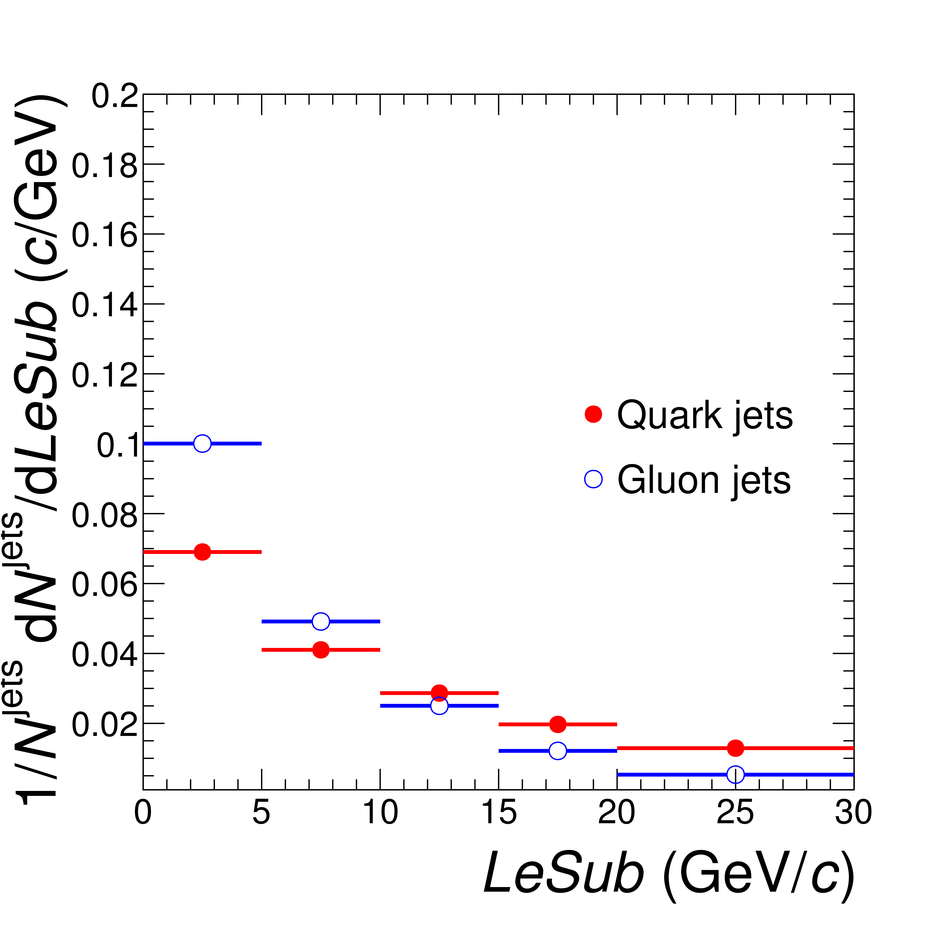## Figure 2

 $g$, $p_{\rm T}D$, and $LeSub$ as obtained from PYTHIA Perugia 2011 simulations of pp collisions at $\sqrt{s}=2.76$\,TeV for three different transverse momentum intervals.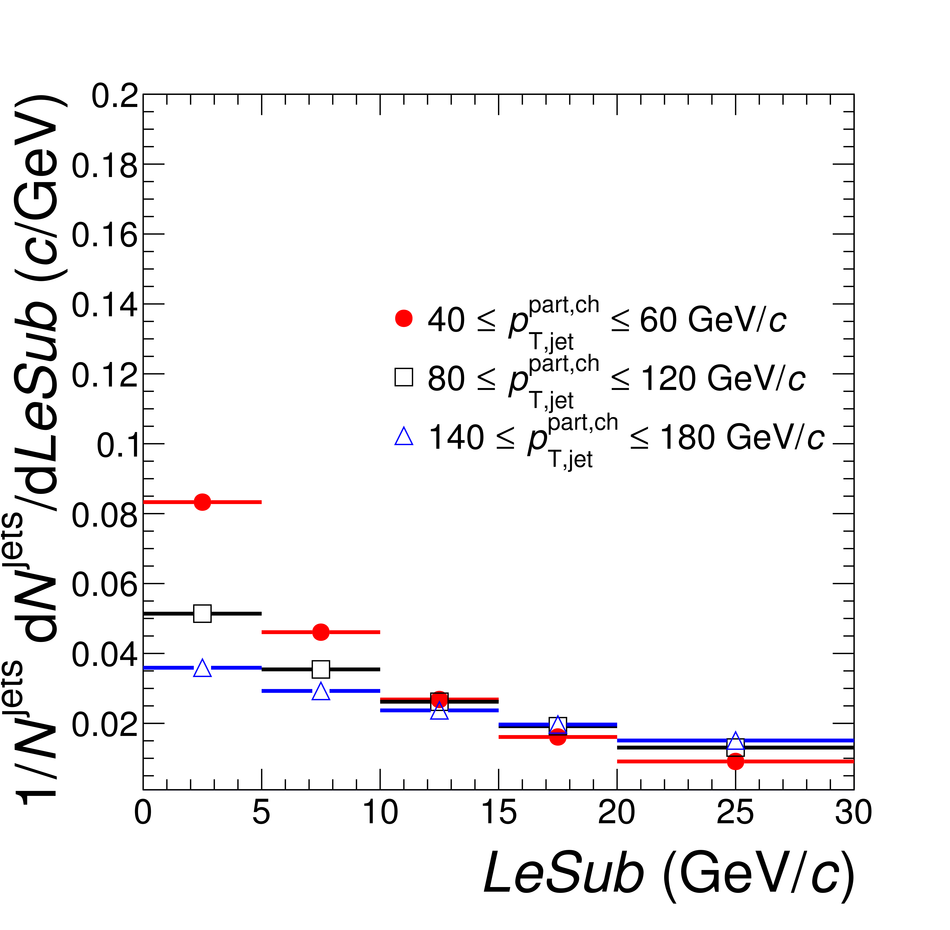## Figure 3

 Background subtraction performance for jet shapes studied with jets from PYTHIA events embedded into real Pb--Pb events, in the background subtracted transverse momentum interval $40 \leq p_{\mathrm{T,jet}}^{\rm{rec,ch}} \leq 60$\,GeV/$c$ for the area derivatives and constituent subtraction methods.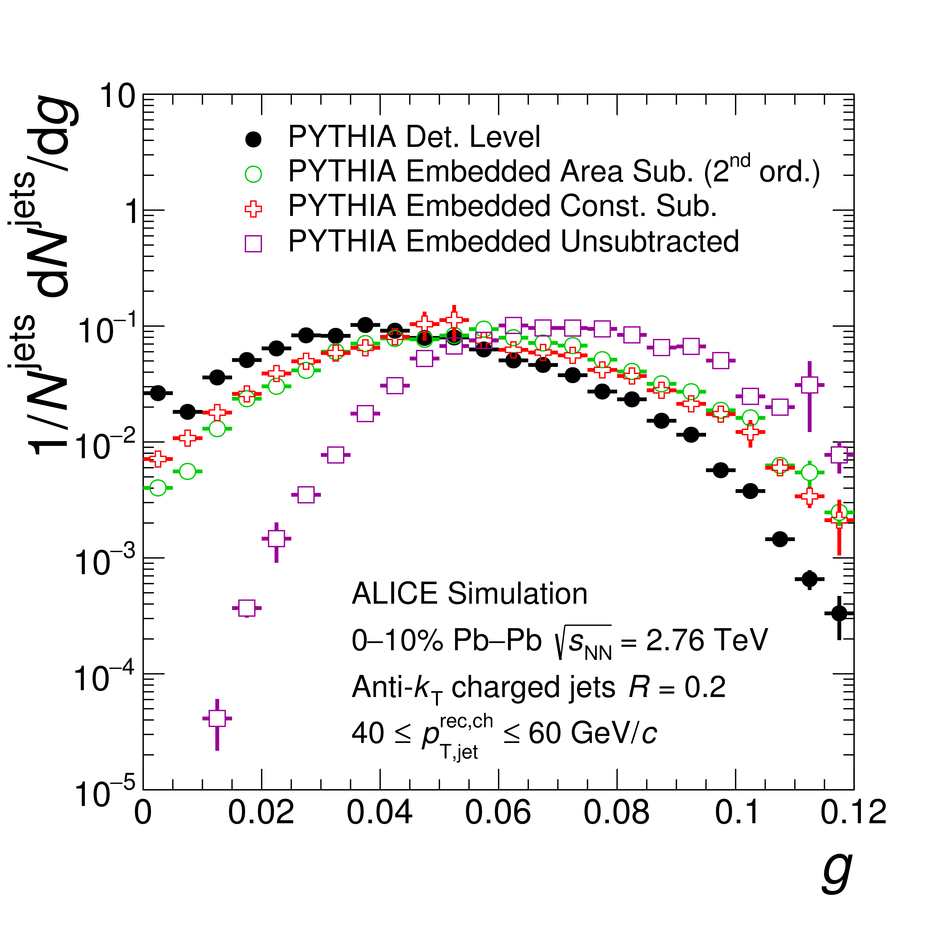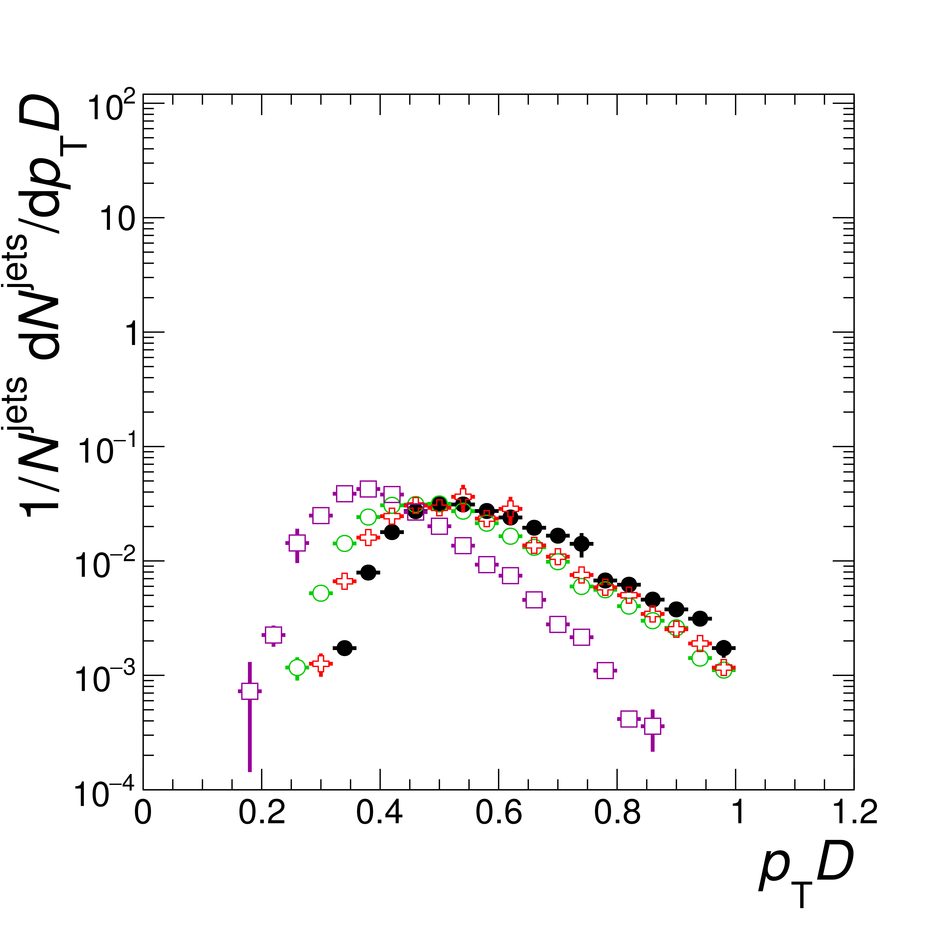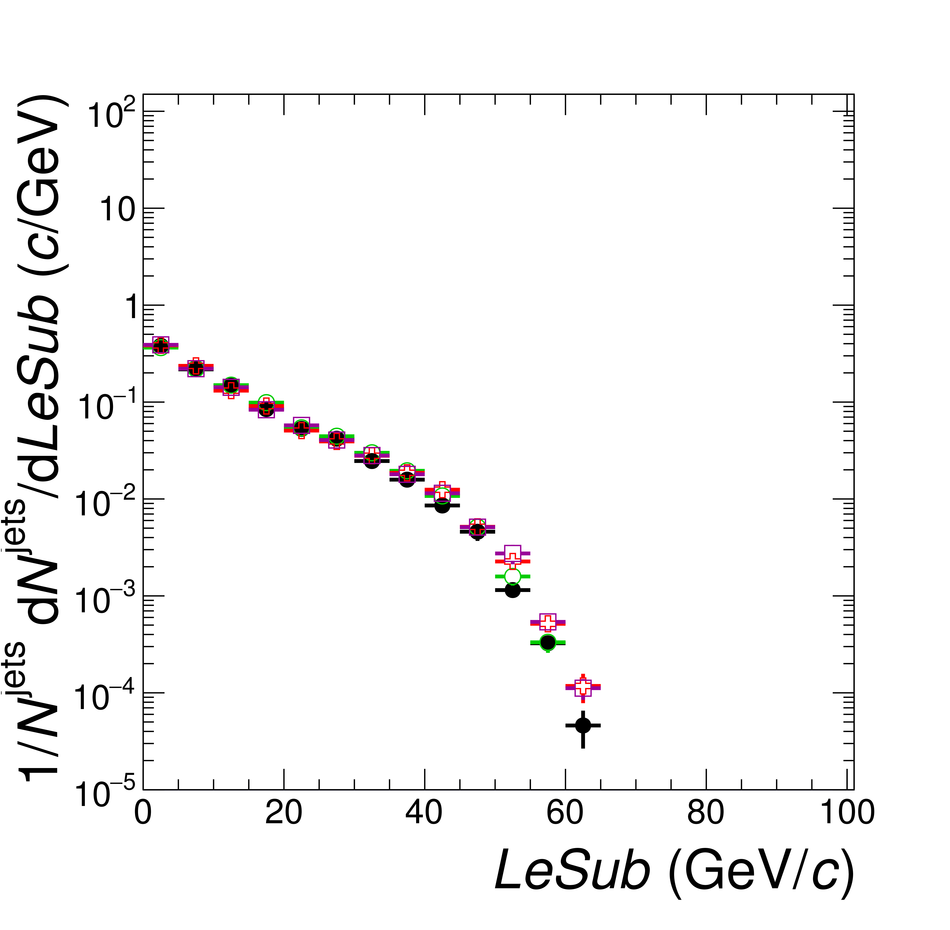## Figure 4

 Left plots show the distributions of residuals for the set of three jet shapes in a given interval of $p_{\rm{T,jet}}^{\rm{part,ch}}$ $40$--$60$ GeV/$c$ using the PYTHIA and PYTHIA embedded simulations. Right plots show the width (quantified as the standard deviation) of the distributions on the left as a function of the values of the shapes at particle level. The black and red curvescorrespond to pp and Pb--Pb simulations, respectively. The line connecting the points on the right is drawn to guide the eye.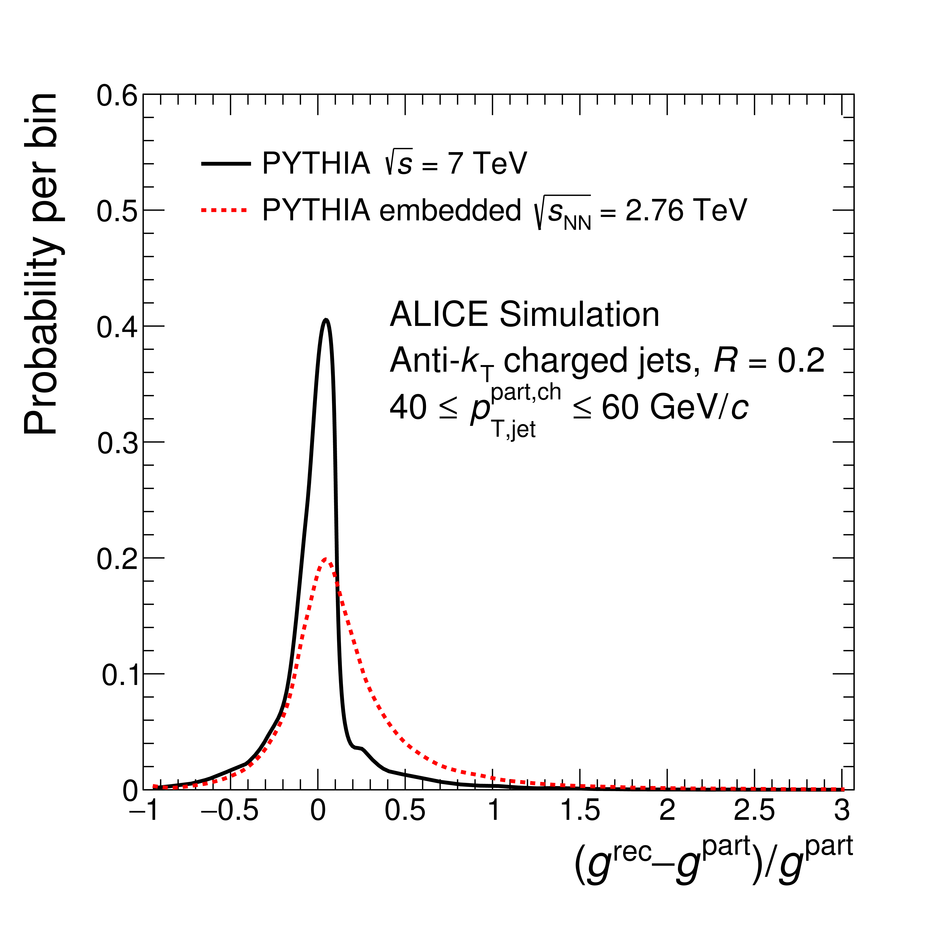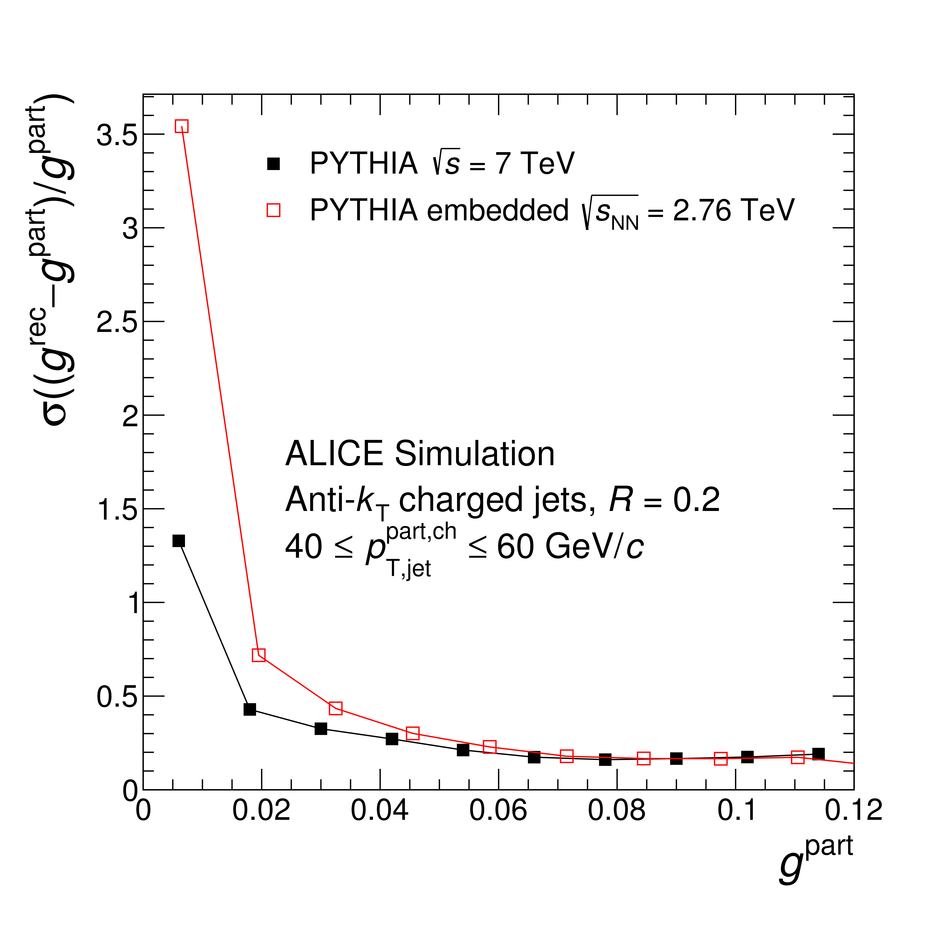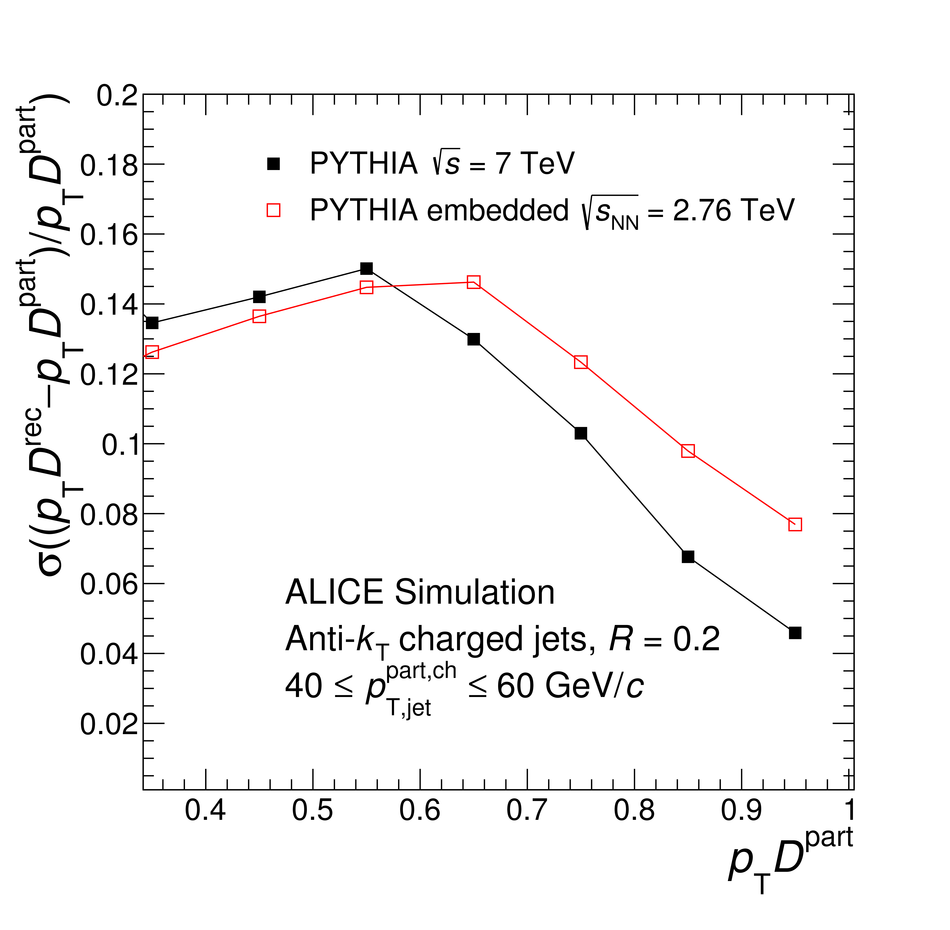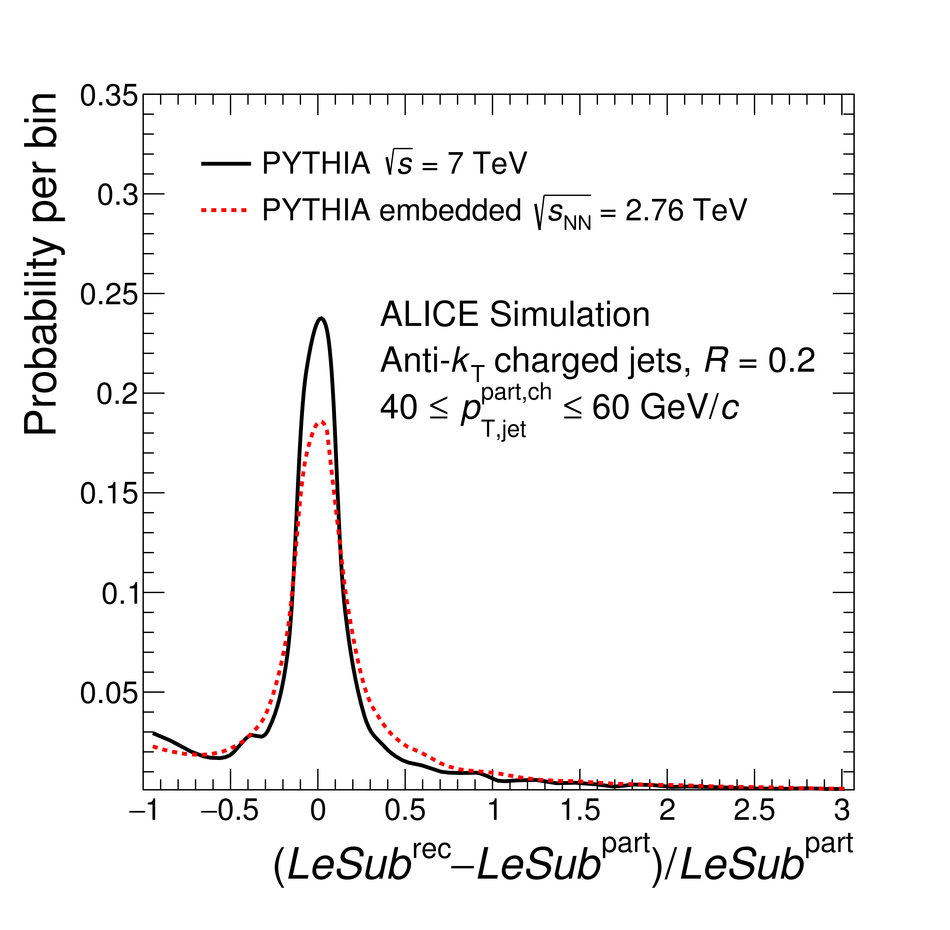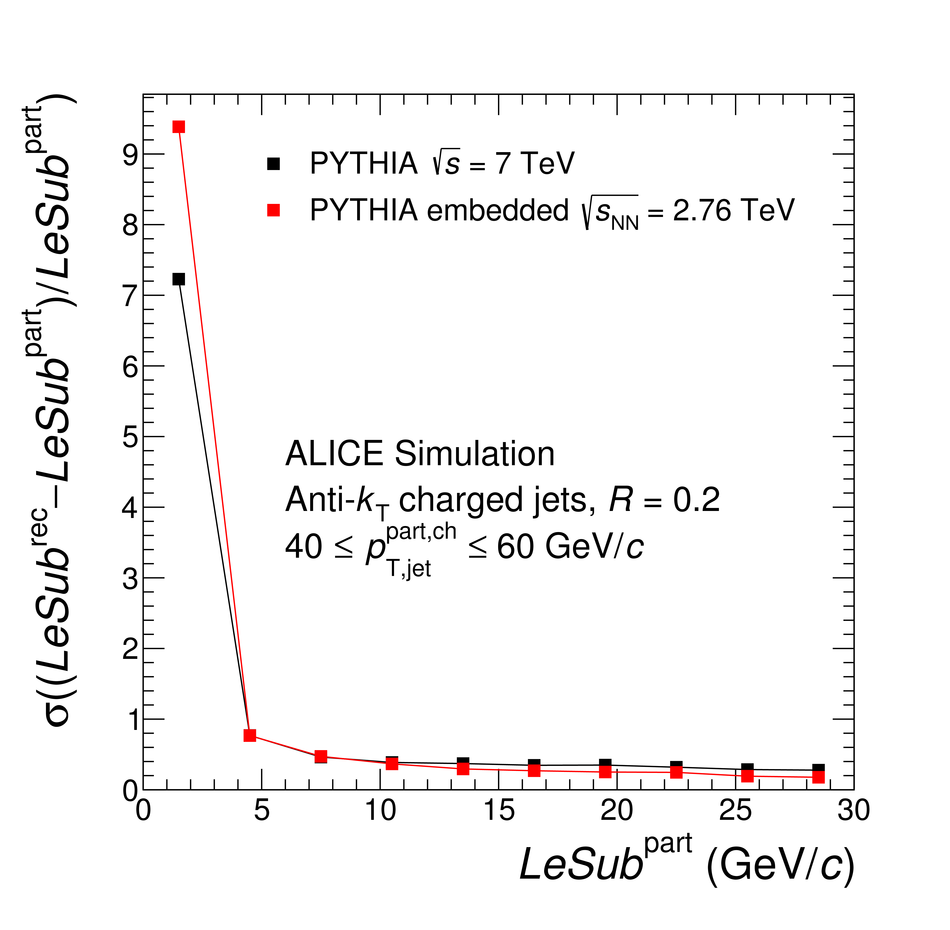## Figure 5

 Fully corrected jet shape distributions measured in pp collisions at $\sqrt{s}=7$\,TeV for $R = 0.2$ in the range of jet $p_{\mathrm{T,jet}}^{\rm ch}$ of $40$--$60$\,GeV$/c$. The results are compared to PYTHIA. The coloured boxes represent the uncertainty on the jet shape (upper panels) and its propagation to the ratio (lower panels)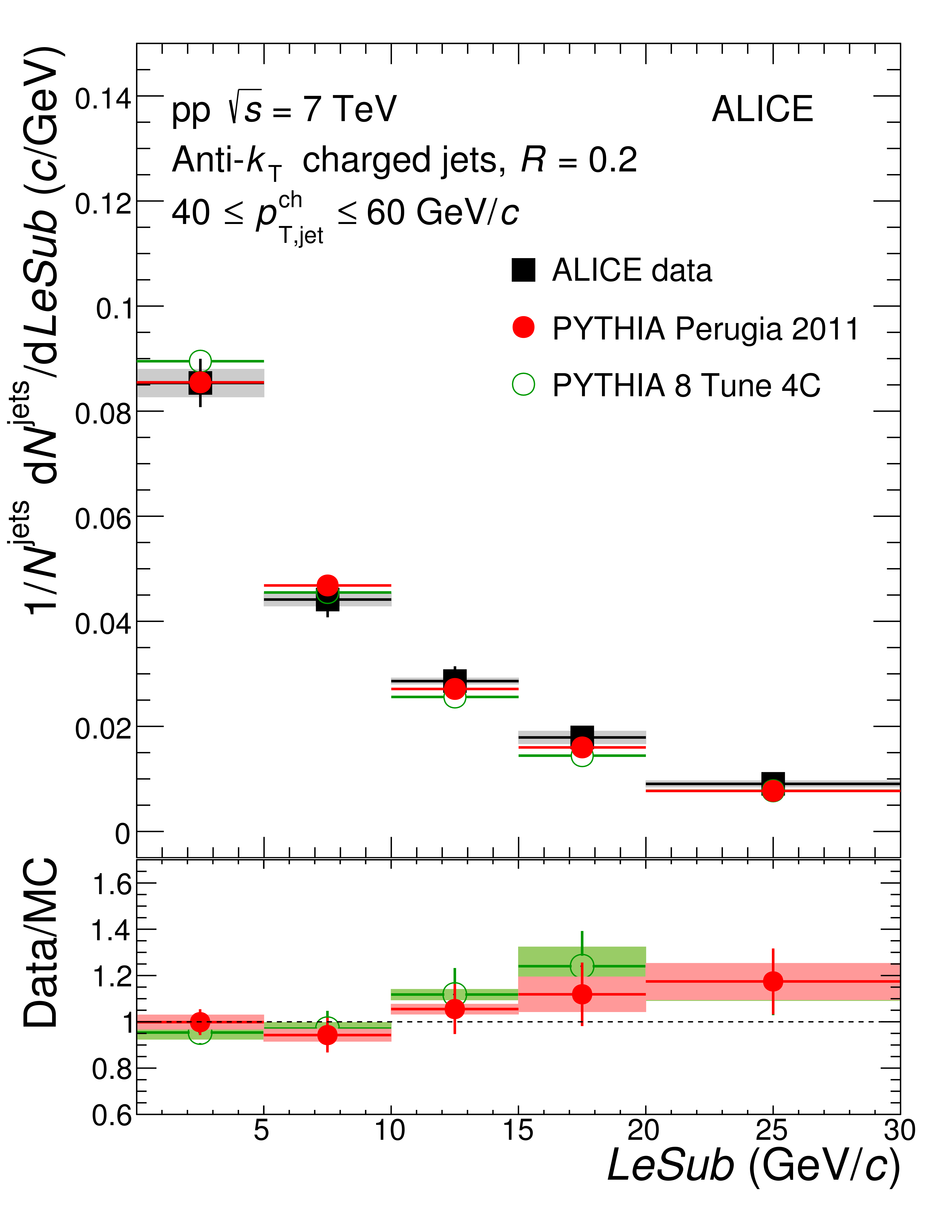## Figure 6

 Fully corrected jet shape distributions in $0$--$10\%$ central Pb--Pb collisions at $\sqrt{s_{\rm NN}} = 2.76$\,TeV for $R = 0.2$ in the range of jet $p_{\mathrm{T,jet}}^{\rm ch}$ of $40$--$60$\,GeV$/c$. The results are compared to PYTHIA. The coloured boxes represent the uncertainty on the jet shape (upper panels) and its propagation to the ratio (lower panels).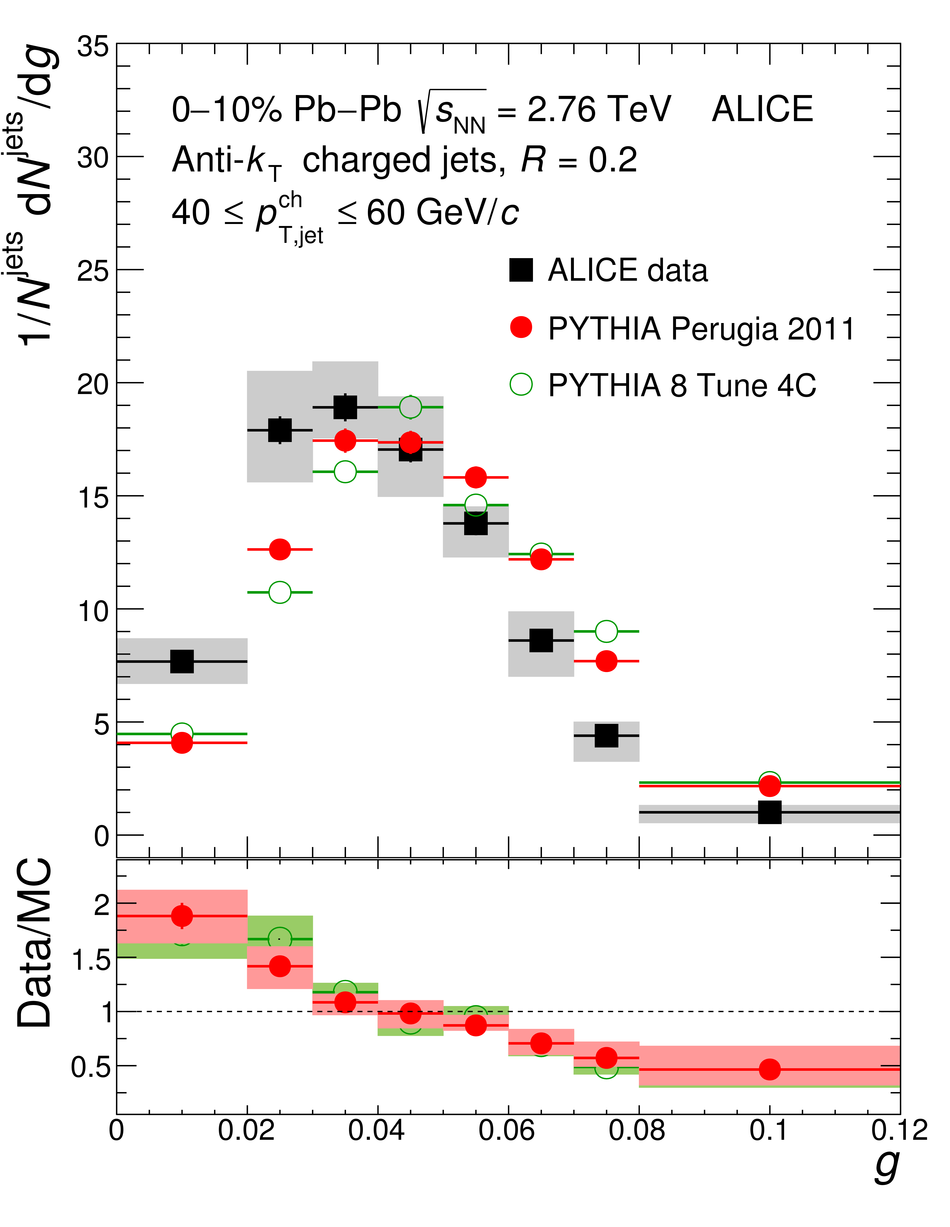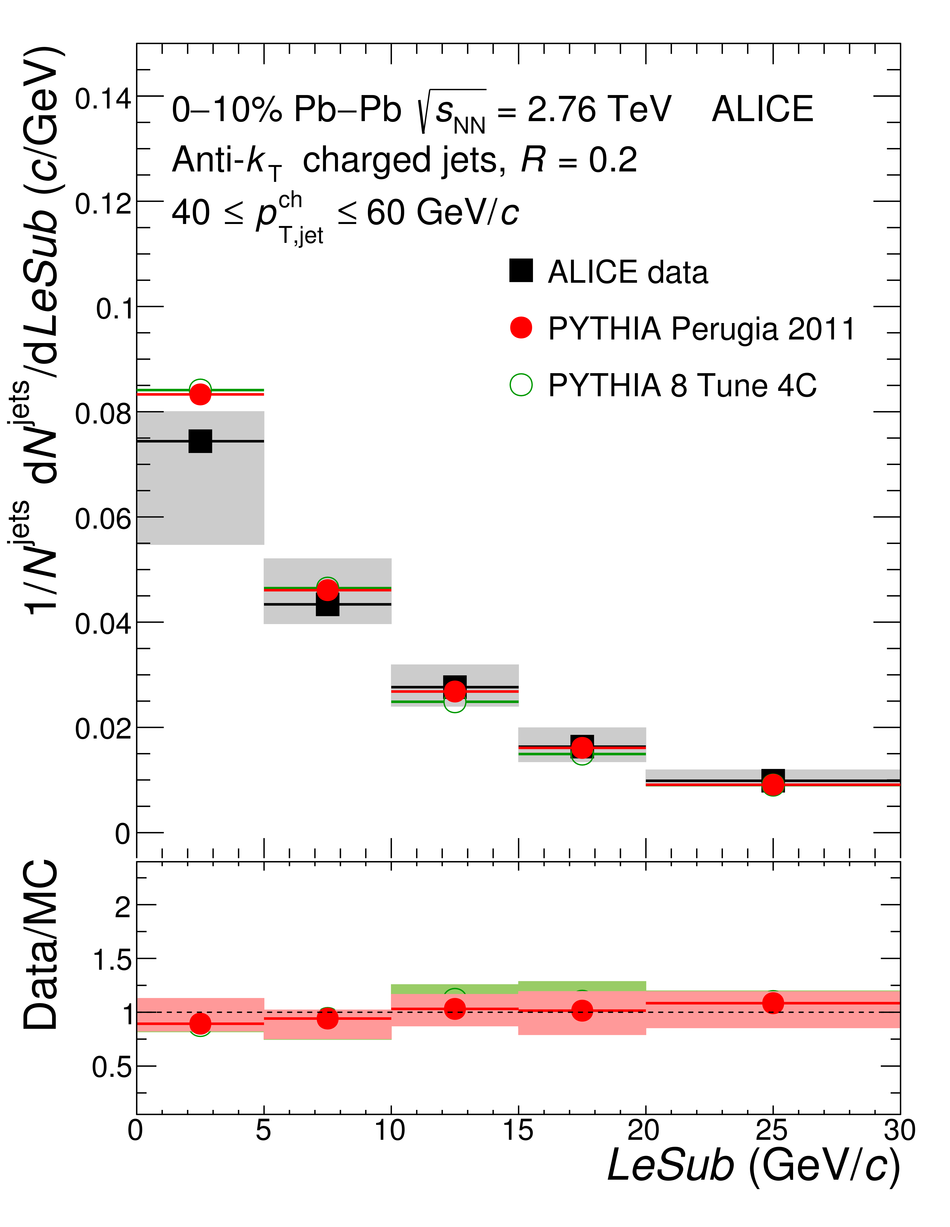## Figure 7

 Jet shape distributions in $0$--$10\%$ central Pb--Pb collisions at $\sqrt{s_{\rm NN}}=2.76$\,TeV for $R = 0.2$ in range of jet $p_{\mathrm{T,jet}}^{\rm ch}$ of $40$--$60$\,GeV$/c$ compared to quark and gluon vacuum generated jet shape distributions. The coloured boxes represent the experimental uncertainty on the jet shapes.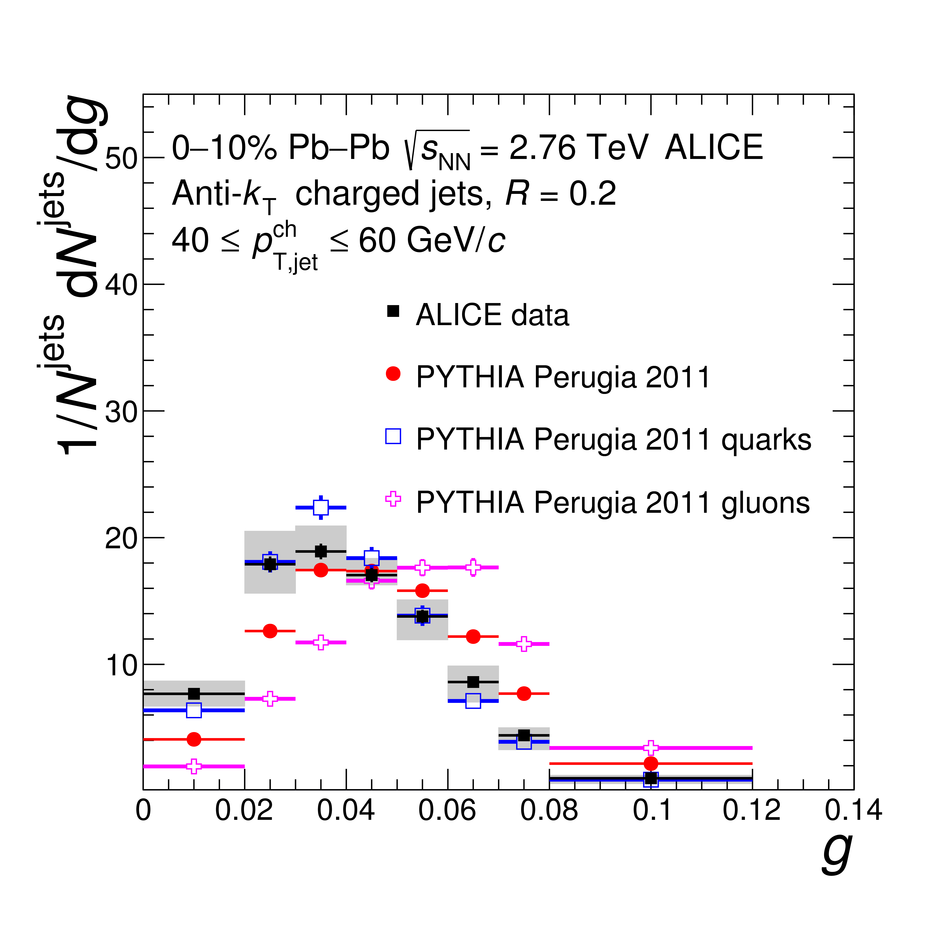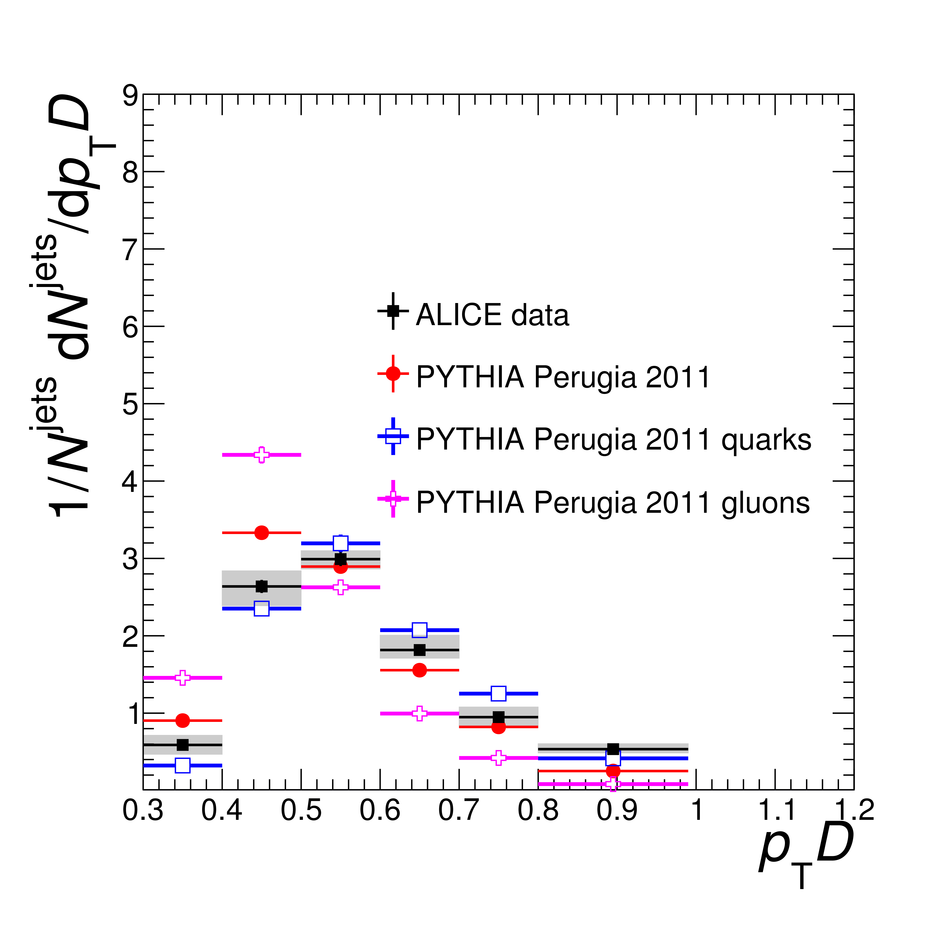## Figure 8

 Jet shape distributions in $0$--$10\%$ central Pb--Pb collisions at $\sqrt{s_{\rm NN}}=2.76$\,TeV for $R = 0.2$ in range of jet $p_{\mathrm{T,jet}}^{\rm ch}$ of $40$--$60$\,GeV$/c$ compared to JEWEL with and without recoils with different subtraction methods. The coloured boxes represent the experimental uncertainty on the jet shapes.